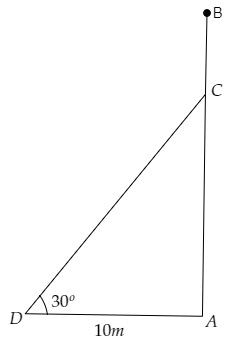# A tree breaks due to the storm and the broken part bends so that the top of the tree touches the ground making an angle of $30^{\circ}$ with the ground. The distance from the foot of the tree to the point where the top touches the ground is 10 metres. Find the height of the tree.

Given:

A tree breaks due to storm and the broken part bends so that the top of the tree touches the ground making an angle of $30^{\circ}$ with the ground.

The distance between the foot of the tree to the point where the top touches the ground is $10 \mathrm{~m}$.

To do:

We have to find the height of the tree.

Solution:Let $AB$ be the original height of the tree and $CD$ be the broken part of the tree that touches the ground at $D$.

Let point $D$ be the point where the top of the broken tree part touches the ground.

From the figure,

$\mathrm{AD}=10 \mathrm{~m}, \angle \mathrm{CDA}=30^{\circ}$

Let the height of the tree from the ground where the tree broke be $\mathrm{CA}=x \mathrm{~m}$ and the height of the broken part be $\mathrm{DC}=y \mathrm{~m}$.

We know that,

$\tan \theta=\frac{\text { Opposite }}{\text { Adjacent }}$

$=\frac{\text { CA }}{DA}$

$\Rightarrow \tan 30^{\circ}=\frac{x}{10}$

$\Rightarrow \frac{1}{\sqrt3}=\frac{x}{10}$

$\Rightarrow x=\frac{10}{\sqrt3} \mathrm{~m}$........(i)

Similarly,

$\cos \theta=\frac{\text { Adjacent }}{\text { Hypotenuse }}$

$=\frac{\text { DA }}{CD}$

$\Rightarrow \cos 30^{\circ}=\frac{10}{y}$

$\Rightarrow \frac{\sqrt3}{2}=\frac{10}{y}$

$\Rightarrow y=\frac{10(2)}{\sqrt3}=\frac{20}{\sqrt3} \mathrm{~m}$

Therefore,

$x+y=\frac{10}{\sqrt3}+\frac{20}{\sqrt3}$

$=\frac{10+20}{\sqrt3}$

$=\frac{30}{\sqrt3}$

$=\frac{30\sqrt3}{\sqrt3 \times \sqrt3}$

$=\frac{30\sqrt3}{3}$

$=10\sqrt3=10(1.73)=17.3 \mathrm{~m}$

Therefore, the height of the tree is $17.3 \mathrm{~m}$.

Updated on: 10-Oct-2022

36 Views